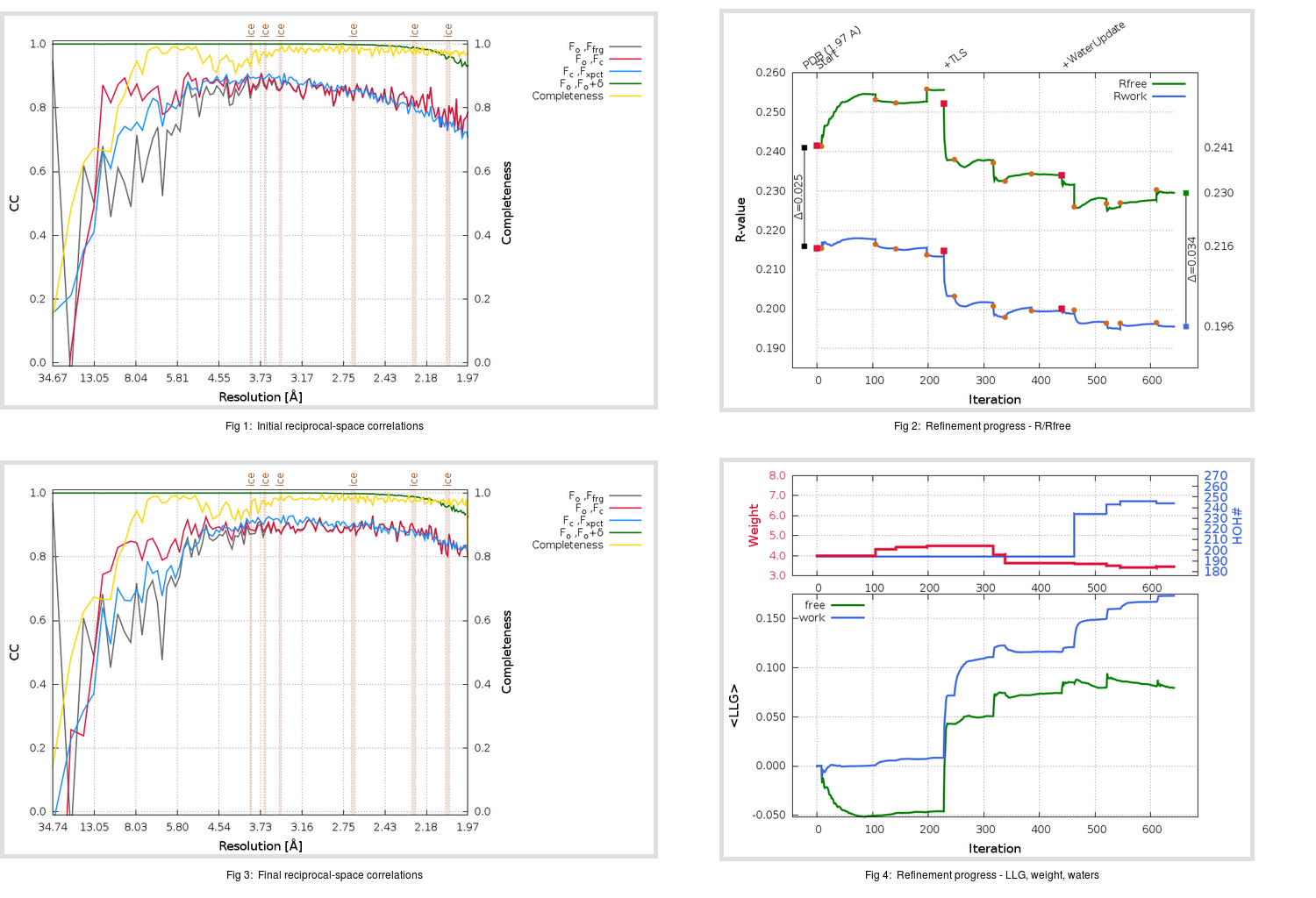Content:

```    Diffraction limits & principal axes of ellipsoid fitted to diffraction cut-off surface:
1.951         0.9393   0.0000   0.3430       0.999 a* + 0.049 c*
1.918         0.0000   1.0000   0.0000       b*
1.884        -0.3430   0.0000   0.9393      -0.573 a* + 0.820 c*
```

## Deposited

` `
 Date deposited Date data collection Resolution R, Rfree 20060511 20051206 1.97 0.2150 0.2410

Molprobity (CCP4 7.0 version) summary:

```Ramachandran outliers =   0.33 %
favored =  96.38 %
Rotamer outliers      =   3.42 %
C-beta deviations     =     0
Clashscore            =   3.60
RMS(bonds)            =   0.0063
RMS(angles)           =   1.29
MolProbity score      =   1.80
Resolution            =   1.97
R-work                =   0.2150
R-free                =   0.2410
```

```Number of waters      =   194

<B> (all atoms) =   41.02 ( sd =    9.86 ) for       2589 non-hydrogen atoms
<B>   (protein) =   40.16 ( sd =    9.25 ) for       2370 non-hydrogen atoms
<B>     (water) =   48.14 ( sd =    9.76 ) for        194 non-hydrogen atoms
<B>    (others) =   67.50 ( sd =    8.23 ) for         25 non-hydrogen atoms

B min/max       (all non-hydrogen atoms) =   21.42 /   84.72
B min/max   (protein non-hydrogen atoms) =   21.42 /   77.91
B min/max     (water non-hydrogen atoms) =   25.98 /   84.72
B min/max     (other non-hydrogen atoms) =   55.67 /   82.60
```

## BUSTER (re-)refinement

` `

Molprobity (CCP4 7.0 version) summary:

```Ramachandran outliers =   0.33 %
favored =  97.37 %
Rotamer outliers      =   5.32 %
C-beta deviations     =     0
Clashscore            =   2.54
RMS(bonds)            =   0.0111
RMS(angles)           =   1.56
MolProbity score      =   1.71
Resolution            =   1.97
R-work                =   0.1956
R-free                =   0.2295
```

```Number of waters      =   244

<B> (all atoms) =   44.56 ( sd =   14.36 ) for       2639 non-hydrogen atoms
<B>   (protein) =   42.62 ( sd =   10.42 ) for       2370 non-hydrogen atoms
<B>     (water) =   53.65 ( sd =   11.49 ) for        244 non-hydrogen atoms
<B>    (others) =  139.57 ( sd =    0.09 ) for         25 non-hydrogen atoms

B min/max       (all non-hydrogen atoms) =   24.78 /  139.69
B min/max   (protein non-hydrogen atoms) =   24.78 /   92.53
B min/max     (water non-hydrogen atoms) =   29.43 /   92.82
B min/max     (other non-hydrogen atoms) =  139.39 /  139.69
```

Refinement progression:Results:

` `
 File Remark 2GZ8_aB_refine.01_03_refine.pdb.gz exact refinement commands are in header 2GZ8_aB_refine.01_03_refine.mtz.gz including original deposited data and several re-refinement map coefficients 2GZ8_aB_refine.01_03_BUSTER_model.cif.gz including any non-standard compound restraints 2GZ8_aB_refine.01_03_BUSTER_refln.cif.gz